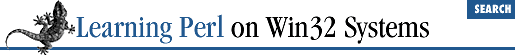home | O'Reilly's CD bookshelfs | FreeBSD | Linux | Cisco | Cisco Exam

### 8.8 Exercises

1. Write a subroutine that takes a numeric value from 1 to 9 as an argument and then returns the English name (such as, ``` one``` , ``` two``` , or ``` nine``` ). If the value is out of range, return the original number as the name instead. Test it with some input data; you'll probably have to write some sort of code to call the subroutine. (Hint: the subroutine should not perform any I/O.)
2. Taking the subroutine from the previous exercise, write a program that takes two numbers and then adds them together, displaying the result as ``` Two``` ``` plus``` ``` two``` ``` equals``` ``` four``` . (Don't forget to capitalize the initial word!)
3. Extend the subroutine to return ``` negative``` ``` nine``` through ``` negative``` ``` one``` and ``` zero``` . Try it in a program.8.7 File-Level my( ) Variables9. Miscellaneous Control Structures